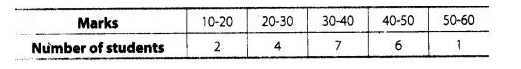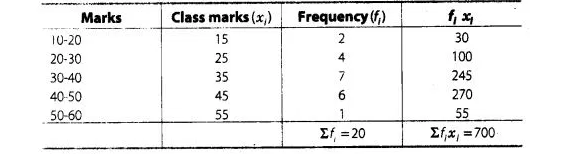# Calculate the mean of the scores

Question:

Calculate the mean of the scores of 20 students in a mathematics testSolution:

We first, find the class mark of each class and then proceed as followsTherefore, $\operatorname{mean}(\bar{x})=\frac{\Sigma f_{i} x_{i}}{\Sigma f_{i}}=\frac{700}{20}=35$

Hence, the mean of scores of 20 students in mathematics test is 35.